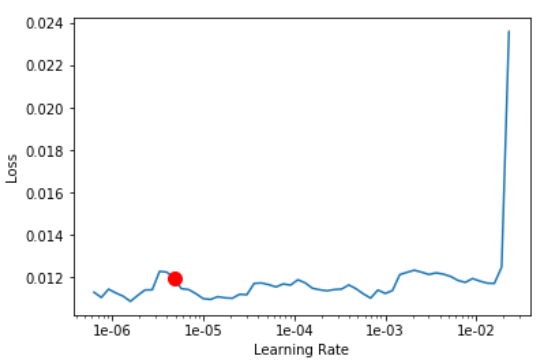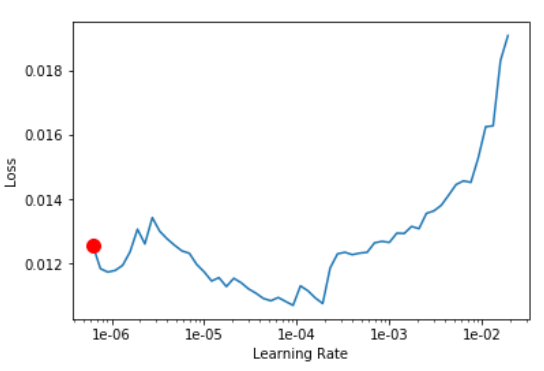# Selecting learning rate in kaggle notebook

(GL) #1

Hi, as far as I understand, currently selection of a learning rate is a manual task, main part of which is visually inspecting the lr plot after calling lr_find().

But in kaggle notebook one cannot submit the result if notebook is run manually. It has to be submitted to be run automatically. So, learning rate(s) have to be hardcoded into the submitted code. Of course, I can also plot the lr_find() and see how close the hardcoded value was from the value I would select if I actually saw the plot.

Actually, I find it that plots (especially for the unfreezed net) really do vary from one run to another, sometimes up to one or even two orders of magnitude. The two examples below are from the two versions of the notebook that differ only in the threshold value used during inference, but totally identical in the data, model, and training code (and I do use seed with split_by_rand_pct):Is there anything that can be done?

0 Likes